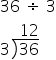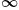Mathematics
Easy

Question

# 36 ÷ 3 = _______

## The correct answer is: 12

###or 36÷ 3 = 12.Quotient = 12 is the answer

When you divide 0 by another number the answer is always 0

When you divide a number by 0 you are not dividing at all , it will undefined for this answer will beWhen you divide by 1, the answer is the same as the number you were dividing

### Related Questions to study#### With Turito Foundation.#### Get an Expert Advice From Turito.